# Vectors and Projectiles

## Part A: Multiple-Multiple Choice

1. Which of the following statements are true of scalars and vectors? List all that are TRUE.

1. A vector quantity always has a direction associated with it.
2. A scalar quantity can have a direction associated with it.
3. Vectors can be added together; scalar quantities cannot.
4. Vectors can be represented by an arrow on a scaled diagram; the length of the arrow represents the vector's magnitude and the direction it points represents the vector's direction.

2. Which of the following quantities are vectors? Include all that apply.

1. distance traveled
2. displacement
3. average speed
4. average velocity
5. instantaneous velocity
6. acceleration

3. Numerical values and directions are stated for a variety of quantities. Which of these statements represent a vector description? Include all that apply.

1. 20 meters, west
2. 9.8 m/s/s
3. 35 mi/hr, south
4. 16 years old
5. 60 minutes
6. 3.5 m/s/s, south
7. -3.5 m/s/s
8. +20 degrees C

4. Which of the following statements are true of vector addition, vector subtraction, and vector addition diagrams? List all that apply.

1. Vectors A, B, and C are added together as A + B + C. If the order in which they are added is changed to C + B + A, then the result would be different.
2. Vectors A, B, and C are added together as A + B + C. If the order in which they are added is reversed to C + B + A, then the result would be a vector with the same magnitude but the opposite direction.
3. When constructing a vector diagram for A + B + C, it is not absolutely necessary that vectors B and C use the same scale that is used by vector A.
4. The resultant in a vector addition diagram always extends from the head of the last vector to the tail of the first vector.
5. If vectors A and B are added at right angles to each other, then one can be sure that the resultant will have a magnitude that is greater than the magnitudes of either one of the individual vectors A and B.
6. If vectors A and B are added at right angles to each other, then one can be sure that the resultant will have a magnitude that is less than the arithmetic sum of the magnitudes of A and B.
7. Vector addition diagrams cannot be used to determine the resultant when there is a vector subtraction operation.

5. Which of the following descriptions of moving objects accurately portray a projectile? List all that apply.

1. an object which is moving through the air and not touching any surface
2. a falling skydiver with an open parachute
3. any object upon which air resistance is negligible
4. a free-falling object
5. an object upon which the only significant force is the force of gravity
6. a falling feather
7. a falling feather in a vacuum chamber
8. a falling feather in a falling vacuum chamber.

6. Which of the following statements are true of projectiles? List all that apply.

1. A projectile is a free-falling object.
2. A projectile experiences negligible or no air resistance.
3. A projectile must be moving in the downward direction.
4. A projectile must be accelerating in the downward direction.
5. A projectile does not have to have horizontal motion.
6. A projectile could begin its projectile motion with a downward velocity.
7. A projectile does not need to be "falling."

7. Which of the following statements are true of the horizontal motion of projectiles? List all that apply.

1. A projectile does not have a horizontal velocity.
2. A projectile with a rightward component of motion will have a rightward component of acceleration.
3. The horizontal velocity of a projectile changes by 9.8 m/s each second.
4. A projectile with a horizontal component of motion will have a constant horizontal velocity.
5. The horizontal velocity of a projectile is 0 m/s at the peak of its trajectory.
6. The horizontal velocity of a projectile is unaffected by the vertical velocity; these two components of motion are independent of each other.
7. The horizontal displacement of a projectile is dependent upon the time of flight and the initial horizontal velocity.
8. The final horizontal velocity of a projectile is always equal to the initial horizontal velocity.
9. As a projectile rises towards the peak of its trajectory, the horizontal velocity will decrease; as it falls from the peak of its trajectory, its horizontal velocity will decrease.
10. Consider a projectile launched from ground level at a fixed launch speed and a variable angle and landing at ground level. The horizontal displacement (i.e., the range) of the projectile will always increase as the angle of launch is increased from 0 degrees to 90 degrees.
11. Consider a projectile launched from ground level at a fixed launch angle and a variable launch speed and landing at ground level. The horizontal displacement (i.e., the range) of the projectile will always increase as the launch speed is increased.

8. Which of the following statements are true of the vertical motion of projectiles? List all that apply.

1. The vertical component of a projectile's velocity is a constant value of 9.8 m/s.
2. The vertical component of a projectile's velocity is constant.
3. The vertical component of a projectile's velocity is changing.
4. The vertical component of a projectile's velocity is changing at a constant rate.
5. A projectile with an upward component of motion will have a upward component of acceleration.
6. A projectile with an downward component of motion will have a downward component of acceleration.
7. The magnitude of the vertical velocity of a projectile changes by 9.8 m/s each second.
8. The vertical velocity of a projectile is 0 m/s at the peak of its trajectory.
9. The vertical velocity of a projectile is unaffected by the horizontal velocity; these two components of motion are independent of each other.
10. The final vertical velocity of a projectile is always equal to the initial vertical velocity.
11. The vertical acceleration of a projectile is 0 m/s/s when it is at the peak of its trajectory.
12. As a projectile rises towards the peak of its trajectory, the vertical acceleration will decrease; as it falls from the peak of its trajectory, its vertical acceleration will decrease.
13. As a projectile rises towards the peak of its trajectory, the vertical acceleration is directed upward; as it falls from the peak of its trajectory, its vertical acceleration is directed downward.
14. The peak height to which a projectile rises above the launch location is dependent upon the initial vertical velocity.
15. As a projectile rises towards the peak of its trajectory, the vertical velocity will decrease; as it falls from the peak of its trajectory, its vertical velocity will decrease.
16. Consider a projectile launched from ground level at a fixed launch speed and a variable angle and landing at ground level. The vertical displacement of the projectile during the first half of its trajectory (i.e., the peak height) will always increase as the angle of launch is increased from 0 degrees to 90 degrees.
17. Consider a projectile launched from ground level at a fixed launch angle and a variable launch speed and landing at ground level. The vertical displacement of the projectile during the first half of its trajectory (i.e., the peak height) will always increase as the launch speed is increased.

9. Which of the following statements are true of the time of flight for a projectile? List all that apply.

1. The time that a projectile is in the air is dependent upon the vertical component of the initial velocity.
2. For a projectile which lands at the same height that it is projected from, the time to rise to the peak is equal to the time to fall from its peak to the original height.
3. For the same upward launch angles, projectiles will stay in the air longer if the initial velocity is increased.
4. Assume that a kicked ball in football is a projectile. If the ball takes 3 seconds to rise to the peak of its trajectory, then it will take 6 seconds to fall from the peak of its trajectory to the ground.

## Part B: Multiple Choice

10. If two displacement vectors of 6 meters and 8 meters (with varying directions) are added together, then the resultant could range anywhere between ___ meters and ___ meters.

 a. 0, 48 b. 1.33, 48 c. 0, 14 d. 2, 14 e. ... nonsense! No such prediction can be made. f. ... nonsense! A prediction can be made but none of these choices are correct.

11. Three vectors are added following the rules of vector addition. A fourth vector is drawn from the tail of the first vector to the head of the last vector. This fourth vector is referred to as ____.

 a. the equilibrant b. the hypotenuse c. the resultant d. a mistake

12. TRUE or FALSE:

The order in which vectors is added will effect the end result.
 a. True b. False

13. Vector A is directed northward and vector B is directed eastward. Which of the following vector addition diagrams best represent the addition of vectors A and B and the subsequent resultant?14. When adding vector B to vector A geometrically (or graphically) using the head to tail method, the resultant is drawn from ____ to the ____.

 a. head of A, tail of B b. tail of A, head of B c. head of B, tail of A d. tail of B, head of A

Use the following vector addition diagrams for Questions #15-#20.15. Which one of the following vector addition equations is shown in Diagram 1?

 a. A + B = C> b. A + C = B c. B + C = A d. B + A = C e. C + B = A g. None of these

16. Which one of the following vector addition equations is shown in Diagram 2?

 a. A + B = C b. A + C = B c. B + C = A d. B + A = C e. C + B = A f. C + A = B g. None of these

17. Which one of the following vector addition equations is shown in Diagram 3?

 a. A + B = C b. A + C = B c. B + C = A d. B + A = C f. C + A = B g. None of these

18. Which one of the following vector addition equations is shown in Diagram 4?

 a. A + B = C b. A + C = B c. B + C = A d. B + A = C e. C + B = A f. C + A = B g. None of these

19. Consider the magnitude and direction of vectors A and B as shown in Diagram 1 above. Which one of the following diagrams would represent B - A = R?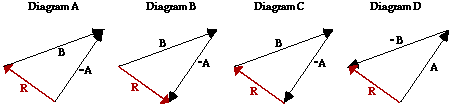20. Consider the magnitude and direction of vectors B and C as shown in Diagram 2 above. Which one of the following diagrams would represent C - B = R?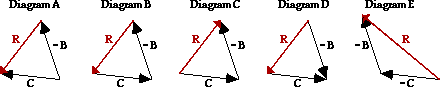21. The vector sum (magnitude only) of 25.0 m, north + 18.0 m, East is ___ m.

 a. 7.00 b. 21.5 c. 30.8 d. 35.8 e. 43.0 f. 54.2 g. 949 h. None of these

22. The vector sum (magnitude only) of 32.0 m, north + 41.0 m, west is ___ m.

 a. 9.00 b. 36.5 c. 38.0 d. 52.0 e. 73.0 f. 128 g. 2.70 x 103 h. None of these

Use the diagram below to answer Questions #23-#28. Each square on the diagram represents a 20-meter x 20-meter area.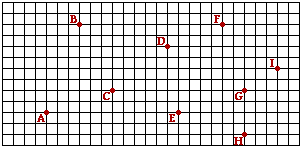23. If a person walks from D to H to G to C, then the distance walked is ____ meters.

 a. 128 b. 180 c. 401 d. 460 e. 480 f. 533 g. 620 h. None of these

24. If a person walks from D to H to G to C, then the magnitude of the displacement is ____ meters.

 a. 128 b. 180 c. 401 d. 460 e. 480 f. 533 g. 620 h. None of these

25. If a person walks from D to H to G to C, then the direction of the displacement is ___ degrees (as measured counterclockwise from East).

 a. 39 b. 51 c. 53 d. 217 e. 219 f. 231 g. 233 h. None of these

26. If a person walks from H to E to C to G, then the distance walked is ____ meters.

 a. 80. b. 240. c. 253 d. 333 e. 493 f. 560. g. 640. h. None of these

27. If a person walks from H to E to C to G, then the magnitude of the displacement is ____ meters.

 a. 80. b. 240. c. 253 d. 333 e. 493 f. 560. g. 640. h. None of these

28. If a person walks from H to E to C to G, then the direction of the displacement is ___ degrees (as measured counterclockwise from East).

 a. 0 b. 18. c. 72 d. 90. e. 108 f. 162 g. 342 h. None of these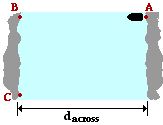Use the following diagram for Questions #29-#33. In the diagram, a riverboat is shown starting at position A on the east bank of a river. The boat heads towards position B (a point directly across the river from A) with a speed of 3.8 m/s. But because of a current with a speed of 1.8 m/s, the boat lands on the west bank of the river at position C, a location downstream from B. The width of the river (dacross) is 86.4 meters.

29. The magnitude of the resultant velocity of the boat is ____ m/s.

 a. 1.8 b. 2.0 c. 3.4 d. 3.8 e. 4.2 f. 5.6 g.11 h. None of these

30. The direction of the resultant velocity of the boat is ____ m/s.

 a. 0 b. 18. c. 712 d. 90. e. 108 f. 162 g. 342 h. None of these

31. The time required for the boat to cross the 86.4 m wide river is ___ seconds.

 a. 4.2 b. 15 c. 21 d. 23 e. 48 f. None of these

32. Location C is the location where the boat ultimately lands on the opposite shore. What is the distance from location B to location C.

 a. 37 b. 41 c. 78 d. 86 e. 96 f. 180 g. 2.0 x 102 h. None of these

33. If the current on a particular day was flowing with two times the velocity, then the time to cross the river would be ____.

 a. two times greater b. one-half as great c. greater, but not two times greater d. less, but not one-half the original time e. the same as it was when the current flowed at 1.8 m/s.

34. An object is undergoing free fall motion. As it falls, the object's ____.

 a. speed increases b. acceleration increases c. both of these d. none of these

35. A football is kicked into the air at an angle of 45 degrees with the horizontal. At the very top of the ball's path, its velocity is _______.

 a. entirely vertical b. entirely horizontal c. both vertical and horizontal d. not enough information given to know.

36. A football is kicked into the air at an angle of 45 degrees with the horizontal. At the very top of the ball's path, its acceleration is _______. (Neglect the effects of air resistance.)

 a. entirely vertical b. entirely horizontal c. both vertical and horizontal d. not enough information given to know.

37. A football is kicked into the air at an angle of 45 degrees with the horizontal. At the very top of the ball's path, the net force acting upon it is _______. (Neglect the effects of air resistance.)

 a. entirely vertical b. entirely horizontal c. both vertical and horizontal d. not enough information given to know.

38. At what point in its path is the horizontal component of the velocity (vx) of a projectile the smallest?

 a. The instant it is thrown. b. Halfway to the top. c. At the top. d. As it nears the top. e. It is the same throughout the path.

39. At what point in its path is the vertical component of the velocity (vy) of a projectile the smallest?

 a. The instant it is thrown. b. Halfway to the top. c. At the top. d. As it nears the top. e. It is the same throughout the path.

40. An airplane that flies at 100 km/h in a 100 km/h hurricane crosswind has a speed (relative to the ground) of ____.

 a. 0 km/h b. 100 km/h c. 141 km/h d. 200 km/h

41. An airplane moves at 141 km/h toward the northeast (45 degrees). What is its component velocity in the northward direction?

 a. 41 km/h b. 100 km/h c. 110 km/h d. 141 km/h

42. Roll a bowling ball off the edge of a table. As it falls, its horizontal component of velocity ___.

 a. decreases b. remains constant c. increases

43. A bullet is fired horizontally and hits the ground in 0.5 seconds. If it had been fired with twice the speed in the same direction, it would have hit the ground in ____. (Assume no air resistance.)

 a. less than 0.5 s. b. more than 0.5 s. c. 0.5 s.

44. A projectile is launched at an angle of 15 degrees above the horizontal and lands down range. For the same speed, what other projection angle would produce the same downrange distance?

 a. 30 degrees. b. 45 degrees. c. 50 degrees. d. 75 degrees e. 90 degrees.

45.   Two projectiles are fired at equal speeds but different angles. One is fired at angle of 30 degrees and the other at 60 degrees. The projectile to hit the ground first will be the one fired at (neglect air resistance) ____.

 a. 30 degrees b. 60 degrees c. both hit at the same time

## Part C: Diagramming and Analysis

46. Express the direction of each of the following vectors in the diagram below.A: ______ B: ______ C: ______ D: ______ E: ______ F: ______

47. In the following diagrams, two vectors are being added and the resultant is drawn. For each diagram, identify which vector is the resultant and write the equation (e.g., A + B = C).

 a.The resultant is vector _____. The equation is ____ + ____ = _____ b.The resultant is vector _____. The equation is ____ + ____ = _____ c.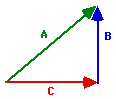The resultant is vector _____. The equation is ____ + ____ = _____ d.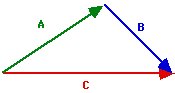The resultant is vector _____. The equation is ____ + ____ = _____

48. Consider the five vectors shown below; they are labeled A, B, C, D and E.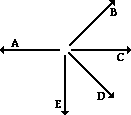Use the stated magnitude and direction of these vectors to sketch a vector addition diagram for the following vector operations and determine the resultant. You only need to construct an approximate sketch; there is no need to do a scaled diagram.

 A + B + C A + C + B A + E + D E + D + C C - D B + E - C

49. A hiker's motion can be described by the following three displacement vectors.

22.0 km, 45 degrees + 16.0 km, 135 degrees + 12.0 km, 270 degrees

(a) What is the distance walked by the hiker?

(b) What is the resulting displacement of the hiker?

50. Solve the following problem using a vector addition diagram.

A hiker hikes for 12.0 km, west. She then makes a turn and hikes in a straight line until she reaches her final destination. If the resultant displacement is 21.0 km at 147 degrees, then what was the displacement (magnitude and direction) of the second leg of the hike?

51. Consider the trajectory diagram shown below for a horizontally launched projectile. On the diagram, draw vector arrows representing the vx and vy velocity components during the course of the motion. The length of the arrows should represent the magnitude of the velocity components. Label each component. (Note that the velocity components are already shown for the first position.)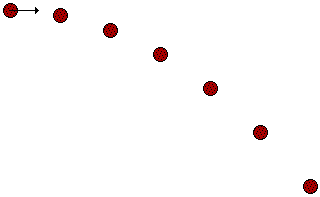52. Consider the trajectory diagram shown below for a non-horizontally launched projectile. On the diagram, draw vector arrows representing the vx and vy velocity components during the course of the motion. The length of the arrows should represent the magnitude of the velocity components. Label each component. (Note that the velocity components are already shown for the first position.)53. Consider the diagram below. The blue path represents the trajectory of a projectile dropped from rest from the top of the path. (Each ball location represents the location after a 1-second interval of time.) The red path represents the trajectory of the same ball thrown horizontally in the absence of gravity. Construct a third path accurately showing the trajectory of a projectile launched with the same horizontal speed as the red ball. Show the location during the first four seconds of motion. Finally, the (x, y) coordinate position of location 1 is (0 m, -5 m) and the (x, y) coordinate position of location 2 is (15 m, 0 m). Determine the (x, y) coordinate positions of the four locations in your trajectory. (Assume g ~10 m/s/s.)54. Consider the map of the United States at the right. Given the scale that 1 cm = 500 km, use a protractor and a ruler to determine the displacement of a traveler for the listed trips. A couple trips include two "legs." Indicate both the magnitude and the direction (using the counterclockwise from East convention) for the resultant displacement vector of the traveler.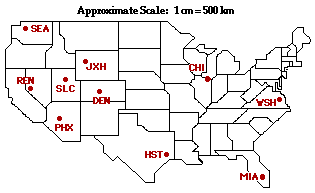Trip Magnitude Direction a. From Chicago (CHI) to Washington, DC (WSH) b. From Chicago (CHI) to Seattle (SEA) c. From Jackson Hole (JXH) to Houston (HST) d. From Denver (DEN) to Phoenix (PHX) to Washington (WSH) e. From Salt Lake City (SLC) to Reno (REN) to Miami (MIA)

55. A cannonball is launched from the top of a 125-meter high cliff with an initial horizontal speed of 20 m/s. The (x, y) coordinate position of the launch location is designated as the (0, 0) position. Determine the (x, y) coordinate positions of the cannonball at 1-second intervals during its path to the ground. Assume g = ~10 m/s/s, down.## Part D: Problem-Solving

56. In the Vector Addition Lab, Anna starts at the classroom door and walks:

• 2.0 meters, West
• 12.0 meters, North,
• 31.0 meters, West,
• 8.0 meters, South
• 3.0 meters, East

Using either a scaled diagram or a calculator, determine the magnitude and direction of Anna's resulting displacement.

57. In a grocery store, a shopper walks 36.7 feet down an aisle. She then turns left and walks 17.0 feet straight ahead. Finally, she turns right and walks 8.2 feet to a final destination. (a) Determine the magnitude of the overall displacement. (b) Determine the direction of the displacement vector relative to the original line of motion.

58. A hiker hikes 12.4 km, south. The hiker then makes a turn towards the southeast and finishes at the final destination. The overall displacement of the two-legged trip is 19.7 km at 309 degrees . Determine the magnitude and direction of the second leg of the trip.

59. A boat heads straight across a river which is 100. meters wide. For the following two combinations of boat velocities and current velocities, determine the resultant velocity, the time required to cross the river, and the distance traveled downstream.

 a. Given: Boat velocity = 10.0 m/s, East River velocity = 4.0 m/s, North Calculate: Resultant Vel. (mag. & dir'n): ________ Time to cross river: _________ Distance traveled downstream: _______ b. Given: Boat velocity = 8.0 m/s, East River velocity = 5.0 m/s, South Calculate: Resultant Vel. (mag. & dir'n): ________ Time to cross river: _________ Distance traveled downstream: _______60. The diagram at the right depicts a horizontally-launched projectile leaving a cliff of height y with a horizontal velocity (vix) and landing a distance x from the base of the cliff. Express your understanding of projectile kinematics by filling in the blanks in the table below. To simplify the calculations, use the approximated value for the acceleration of gravity of -10 m/s/s.

 vix (m/s) y (m) t (s) x (m) a. 15.0 m/s 20.0 m ______ ______ b. 15.0 m/s ______ 3.00 s ______ c. ______ 45.0 m ______ 45.0 m d. ______ ______ 2.50 s 30.0 m e. ______ 74.0 m ______ 66.0 m

61. The launch velocity and angle is given for three different projectiles. Use trigonometric functions to resolve the velocity vectors into horizontal and vertical velocity components. Then use kinematic equations to determine the time that the projectile is in the air, the height to which it travels (when it is at its peak), and the horizontal distance that it travels. (To simplify the calculations, use an acceleration of gravity value of -10 m/s/s.)

 a. Given: Launch Vel. = 30.0 m/s Launch angle = 30.0 degrees b. Given: Launch Vel. = 30.0 m/s Launch angle = 45.0 degrees c. Given: Launch Vel. = 30.0 m/s Launch angle = 50.0 degrees Calculate: vix = __________ viy = ___________ tup = ___________ ttotal = ___________ y at peak = ___________ x = ___________ Calculate: vix = __________ viy = ___________ tup = ___________ ttotal = ___________ y at peak = ___________ x = ___________ Calculate: vix = __________ viy = ___________ tup = ___________ ttotal = ___________ y at peak = ___________ x = ___________

62. If a projectile is launched horizontally with a speed of 12.0 m/s from the top of a 24.6-meter high building. Determine the horizontal displacement of the projectile.

63. A projectile is launched with an initial speed of 21.8 m/s at an angle of 35.0-degrees above the horizontal.

(a) Determine the time of flight of the projectile.

(b) Determine the peak height of the projectile.

(c) Determine the horizontal displacement of the projectile.

64. A projectile is launched horizontally from the top of a 45.2-meter high cliff and lands a distance of 17.6 meters from the base of the cliff. Determine the magnitude of the launch velocity.

65. Two physics students stand on the top of their 3.29-meter second-story deck and launch a water balloon from a homemade winger. The balloon is launched upward at a speed of 45.2 m/s and an angle of 39.1 degrees. The balloon lands in a retention pond whose surface is 2.92 meters below grade. Determine the horizontal distance from launch location to landing location.

66. A place kicker kicks a football from 39.6 meters from the goal posts. The kick leaves the ground with a speed of 24.8 m/s at an angle of 49.6 degrees. The goal posts are 3.10-meters high.

(a) Determine the amount by which the kick clears the goal posts.

(b) For this given launch velocity, what is the longest field goal (in yards) which could have been kicked? Assume that the football hits the horizontal crossbar of the posts and bounces through. Given: 1.00 meter = 3.28 feet.

67. An airplane starts at Point A and flies 210. km at 311 degrees to Point B. The plane then flies 179 km at 109 degrees to Point C. Finally, the plane flies 228 km at 29 degrees to Point D. Determine the resulting displacement (magnitude and direction) from Points A to D.

68. Sammy Sosa clubs a homerun which sails 421 feet and lands on an apartment balcony located a vertical distance of 59.0 feet above the level of the ball-bat contact location. An observer times the flight to the balcony to take 3.40 seconds.

(a) Determine the velocity (magnitude and angle) at which the ball leaves the bat.

(b) Determine the speed of the ball (in miles/hour) when it lands in the bleachers.

Given: 1.00 m/s = 2.24 mi/hr; 1.00 meter = 3.28 feet.

69. An unfortunate accident occurred on the toll way. A driver accidentally passed through a faulty barricade on a bridge (quite unfortunately). and landed in a pile of hay (quite fortunately). Measurements at the accident scene reveal that the driver plunged a vertical distance of 8.26 meters. The car carried a horizontal distance of 42.1 meters from the location where it left the bridge. If the driver was in a 65 mi/hr speed zone, then determine the amount by which the driver was exceeding the speed limit at the time of the accident. Assume that the contact with the barricade did not slow the car down. (1.00 m/s = 2.24 mi/hr)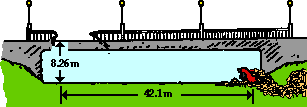70. Cupid wishes to shoot an arrow through the open window of a tall building. The window is 32.8 meters above the ground and Cupid stands 63.6 meters from the base of the building. If Cupid aims the arrow at an angle of 51.5 degrees above the horizontal, with what minimum speed must he fire the arrow in order for it to enter the window?

71. In a Physics demonstration, a projectile is launched from a height of 1.23 m above the ground with a speed of 10.6 m/s at an angle of 30.0 degrees above the horizontal.

(a) What horizontal distance from the launch location will the projectile land?

(b) With what speed does the projectile land?

72. A car is parked on a cliff overlooking the sea. The cliff is inclined at an angle of 29.0 degrees below the horizontal. The negligent driver leaves the car in neutral and it begins rolling from rest towards the cliff's edge with an acceleration 4.50 m/s/s. The car moves a linear distance of 57.2 m to the edge of the cliff before plunging into the ocean below. The cliff is 42.2 m above the sea.

(a) Find the speed (in m/s) of the car the moment it leaves the cliff.

(b) Find the time (in seconds) it takes the car to drop to the water below the edge of the cliff.

(c) Find the position (in meters) of the car relative to the base of the cliff when it lands in the sea.

## Navigate to:

Review Session Home - Topic Listing

Vectors and Projectiles - Home || Printable Version || Questions and Links

Answers to Questions:  All  ||  #1-9  ||  #10-45  ||  #46-55  ||  #56-72

## You Might Also Like ...

Users of The Review Session are often looking for learning resources that provide them with practice and review opportunities that include built-in feedback and instruction. If that is what you're looking for, then you might also like the following:

The Calculator Pad includes physics word problems organized by topic. Each problem is accompanied by a pop-up answer and an audio file that explains the details of how to approach and solve the problem. It's a perfect resource for those wishing to improve their problem-solving skills.

Visit: The Calculator Pad Home | Calculator Pad - Vectors and Projectiles

2. Minds On Physics the App Series

Minds On Physics the App ("MOP the App") is a series of interactive questioning modules for the student that is serious about improving their conceptual understanding of physics. Each module of the series covers a different topic and is further broken down into sub-topics. A "MOP experience" will provide a learner with challenging questions, feedback, and question-specific help in the context of a game-like environment. It is available for phones, tablets, Chromebooks, and Macintosh computers. It's a perfect resource for those wishing to refine their conceptual reasoning abilities. Part 1 of the series includes Vectors and Projectiles.

Visit: MOP the App Home || MOP the App - Part 1